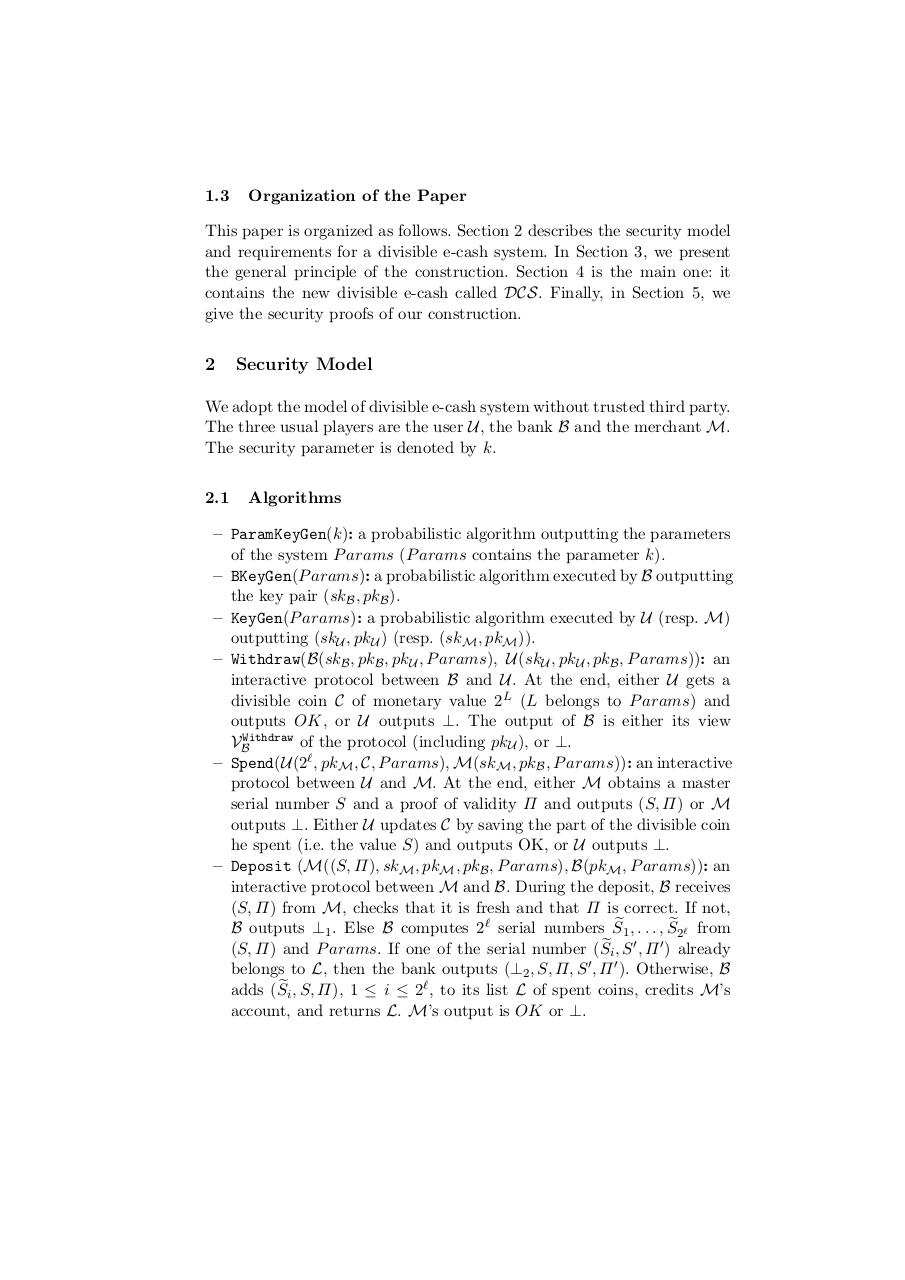# e cash satoshi for reddit.pdfPage 1 2 34518

#### Text preview

1.3

Organization of the Paper

This paper is organized as follows. Section 2 describes the security model
and requirements for a divisible e-cash system. In Section 3, we present
the general principle of the construction. Section 4 is the main one: it
contains the new divisible e-cash called DCS. Finally, in Section 5, we
give the security proofs of our construction.

2

Security Model

We adopt the model of divisible e-cash system without trusted third party.
The three usual players are the user U, the bank B and the merchant M.
The security parameter is denoted by k.
2.1

Algorithms

– ParamKeyGen(k): a probabilistic algorithm outputting the parameters
of the system P arams (P arams contains the parameter k).
– BKeyGen(P arams): a probabilistic algorithm executed by B outputting
the key pair (skB , pkB ).
– KeyGen(P arams): a probabilistic algorithm executed by U (resp. M)
outputting (skU , pkU ) (resp. (skM , pkM )).
– Withdraw(B(skB , pkB , pkU , P arams), U(skU , pkU , pkB , P arams)): an
interactive protocol between B and U. At the end, either U gets a
divisible coin C of monetary value 2L (L belongs to P arams) and
outputs OK, or U outputs ⊥. The output of B is either its view
VBWithdraw of the protocol (including pkU ), or ⊥.
– Spend(U(2ℓ , pkM , C, P arams), M(skM , pkB , P arams)): an interactive
protocol between U and M. At the end, either M obtains a master
serial number S and a proof of validity Π and outputs (S, Π) or M
outputs ⊥. Either U updates C by saving the part of the divisible coin
he spent (i.e. the value S) and outputs OK, or U outputs ⊥.
– Deposit (M((S, Π), skM , pkM , pkB , P arams), B(pkM , P arams)): an
interactive protocol between M and B. During the deposit, B receives
(S, Π) from M, checks that it is fresh and that Π is correct. If not,
B outputs ⊥1 . Else B computes 2ℓ serial numbers Se1 , . . . , Se2ℓ from
(S, Π) and P arams. If one of the serial number (Sei , S ′ , Π ′ ) already
belongs to L, then the bank outputs (⊥2 , S, Π, S ′ , Π ′ ). Otherwise, B
adds (Sei , S, Π), 1 ≤ i ≤ 2ℓ , to its list L of spent coins, credits M’s
account, and returns L. M’s output is OK or ⊥.